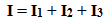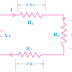The connection between electrical or electronic components is designed in many ways to get the desired output. The electrical or electronic component can be connected in three ways that are,

(1) They can be connected in series only
(2) They can be connected in parallel only
(3) They can be connected in both series and parallel combination.

## Series Circuit

Definition: When electrical or electronic components are connected in back to back or in series, then the circuit created by them is called Series Circuit.

### Properties of Series Circuit:

(1) All components of a series circuit are connected along a single conductive path.
(2) The flow of current through each component is the same.
(3) If the resistance of the components is different then the voltage drop across them will be different.
(4) In a series circuit, the summation of the voltage drop of each component is equal to the source or supply voltage.
(5) The series circuit obeys the Kirchhoff's voltage law.
(6) In a series circuit, the total resistance can be found by simply adding the resistance of each component.

### DC Series Circuit:

In the above figure, you can see here three resistors are connected in series.
The total resistance or equivalent resistance can be found very easily. By adding all resistances we can get the total resistance.
So the total resistance will be,As all resistors are connected in series, so the same current will flow through each resistor which can be found as below,The voltage drop across each resistor is shown in the figure. We know that the summation of the voltage drop across each resistor is equal to the supply voltage.
So,Example of Series Circuit: The Rice Lights which are used for decoration at Diwali

## Parallel Circuit

When electrical or electronic components are connected parallelly that means top ends are connected together and bottom ends are connected together then the circuit is called Parallel Circuit.

### Properties of Parallel Circuit:

(1) The voltage drop across each component is equal to the   Source voltage or supply voltage.
(2) The current flowing through each component will be different.
(3) The flow of current through the components depends upon their internal resistance.
(4) The summation of the flow of current through each component is equal to the total current.
(5) Parallel Circuit obeys the Kirchhoff's current law.

### DC Parallel Circuit:

As you see in the above figure, three resistors are connected in parallel that means top ends of each resistor are connected together and the bottom ends of each resistor are connected together.

As I told you, here different current flows through each resistor.
The total current,The voltage drop across each resistor is equal to the source voltage so we can write,The equivalent resistance of the circuit,Example of a Parallel Circuit: In our house, offices all loads are connected in parallel.

Let's see the difference between Series and Parallel Circuit at a glance,

### Series Circuit VS Parallel Circuit:

 SL NO. Series Circuit Parallel Circuit 1. Here tail of a component is connected to the head of the next component. Here heads of each component are directly connected together and tails of each component are directly connected together. 2. The current flowing through each component is the same. The current flowing through each component is not the same, it depends upon the resistance of the component. 3. The voltage drop across each component is not the same, it depends upon the resistance of the components. The voltage drop across each component is the same which is equal to the source voltage. 4. Series Circuit obeys the Kirchhoff's voltage law. Parallel Circuit obeys the Kirchhoff's current law. 5. In the series circuit, if one component is damaged then other components will not get supply. That means all components are depended on each other. In the parallel circuit, if one component is damaged then other components will not be affected, that means here all components are independent on each other.

### Series and Parallel Combination Circuit

Here resistors are connected in series and parallel combination. In the above circuit, R2 and R3 are connected in parallel. So at first, we should find the equivalent resistance of R2 and R3. Then R1, R4, and the equivalent resistance are to be added to find the total resistance of the circuit.
So the total resistance of the circuit can be found by the below equation,Example of Series and Parallel Combination Circuit: Mostly electronics circuits are designed with series and parallel combination.

Let's solve some problems,

Q.1. Three resistors 5ohm, 9ohm, 10ohm are connected in series. If the circuit is connected across the 12V DC supply then what will be the voltage drop across the 9ohm resistor?

Ans: First of all, let's draw the circuit.
It is a series circuit so the total resistance will be, R = 5+9+10 = 24ohm
Supply Voltage = 12V
So the current, I = V/R = 12/24 = 0.5 amps
Now, the voltage drop across the 9ohm resistor is, 0.5×9 = 4.5V

Q.2. Three resistors 2ohm, 6ohm, 4ohm are connected in parallel. If the circuit is connected across the 24V DC supply, how much current will flow through the 4ohm resistor? Also, find the total current of the Circuit.

Ans: Let's draw the circuit,

The flow of current through the 4ohm resistor is, 24/4 = 6 amps
The equivalent resistance of the circuit is,
1/R = 1/2 + 1/6 + 1/4
So, R = 1.1ohm (approximately)

So the total current of the circuit is, I = 24/1.1 = 21.81 amps

Thank you for visiting the website. keep visiting for more updates.

Series Circuit and Parallel Circuit ExplanationReviewed by Author on 3/04/2019 Rating: 5